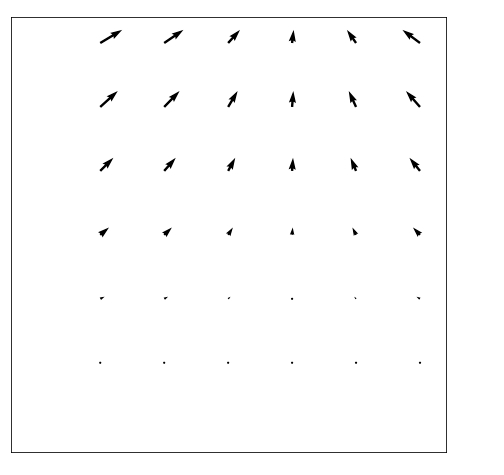# Explain how a quiver plot can be built using Matplotlib Python?

Matplotlib is a popular Python package that is used for data visualization. Visualizing data is an important steps since it helps understand what is going on in the data without actually looking at the numbers and performing complicated computations. It helps in communicating the quantitative insights to the audience effectively.

Matplotlib is used to create 2 dimensional plots with the data. It comes with an object oriented API that helps in embedding the plots in Python applications. Matplotlib can be used with IPython shells, Jupyter notebook, Spyder IDE and so on.

It is written in Python. It is created using Numpy, which is the Numerical Python package in Python.

Python can be installed on Windows using the below command −

pip install matplotlib

The dependencies of Matplotlib are −

Python ( greater than or equal to version 3.4)
NumPy
Setuptools
Pyparsing
Libpng
Pytz
Free type
Six
Cycler
Dateutil

A quiver plot is used to display velocity vectors as arrays that have components (u , v) at points (x , y) respectively. It can be created using the below command −

quiver(x,y,u,v, color)


Here, ‘x’, ‘y’, ‘u’ and ‘v’ can be coordinates of 1−D or 2−D sequence of data

Let us understand how Matplotlib can be used to create quiver plot −

import numpy as np
import matplotlib.pyplot as plt

x = np.arange(0, 5.4, 0.5)
y = np.arange(0, 5.4, 0.5)

X, Y = np.meshgrid(x, y)
u = np.cos(X)*Y
v = np.sin(Y)*Y

fig, ax = plt.subplots(figsize =(12, 8))
ax.quiver(X, Y, u, v)

ax.xaxis.set_ticks([])
ax.yaxis.set_ticks([])
ax.axis([−0.7, 2.7, −0.7, 2.7])
ax.set_aspect('equal')

plt.show()

## Output## Explanation

• The required packages are imported, and aliased.

• The data is generated using NumPy library.

• The sine and cos functions are defined.

• The size of the plot is defined.

• The ‘quiver’ function present in Matplotlib is called.

• The ‘show’ function is used to display the plot.